Download Presentation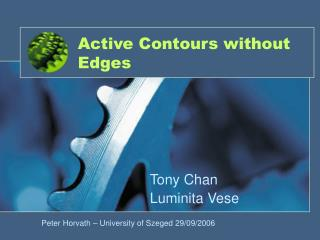Active Contours without Edges

# Active Contours without Edges - PowerPoint PPT Presentation

Active Contours without Edges. Tony Chan Luminita Vese. Peter Horvath – University of Szeged 29/09/2006 . Introduction. Variational approach The main problem is to minimise an integral functional (e.g.): In the case f: , f’=0 gives the extremum(s)I am the owner, or an agent authorized to act on behalf of the owner, of the copyrighted work described.
Download Presentation## Active Contours without Edges

An Image/Link below is provided (as is) to download presentation

Download Policy: Content on the Website is provided to you AS IS for your information and personal use and may not be sold / licensed / shared on other websites without getting consent from its author.While downloading, if for some reason you are not able to download a presentation, the publisher may have deleted the file from their server.

- - - - - - - - - - - - - - - - - - - - - - - - - - E N D - - - - - - - - - - - - - - - - - - - - - - - - - -
Presentation Transcript### Active Contours without Edges

Tony Chan

Luminita Vese

Peter Horvath – University of Szeged 29/09/2006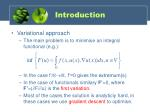Introduction
• Variational approach
• The main problem is to minimise an integral functional (e.g.):
• In the case f:, f’=0 gives the extremum(s)
• In the case of functionals similary F’=0, where F’=(F/u) is the first variation.
• Most of the cases the solution is analyticly hard, in these cases we use gradient descent to optimise.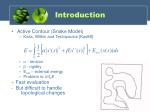Introduction
• Active Contour (Snake Model)
• Kass, Witkin and Terzopoulos [Kas88]
•  - tension
•  - rigidity
• Eext – external energy
• Problem is: infxE

+ Fast evaluation

- But difficult to handle topological changes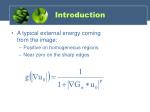Introduction
• A typical external energy coming from the image:
• Positive on homogeneous regions
• Near zero on the sharp edges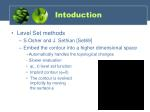Intoduction
• Level Set methods
• S.Osher and J. Sethian [Set89]
• Embed the contour into a higher dimensional space

+Automatically handles the topological changes

- Slower evaluation

• (., t) level set function
• Implicit contour (=0)
• The contour is evolved implicitly by moving the surface 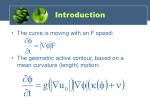Introduction
• The curve is moving with an F speed:
• The geometric active contour, based on a mean curvature (length) motion: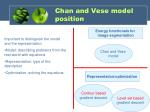Chan and Vese model position

Energy functionals for image segmentation

• Important to distinguish the model and the representation
• Model: describing problems from the real world with equations
• Representation: type of the description
• Optimization: solving the equations

Chan and Vese model

Representation/optimization

Level set basedgradient descent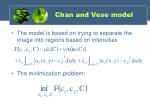Chan and Vese model
• The model is based on trying to separate the image into regions based on intensities
• The minimization problem:Chan and Vese model
• c1 and c2 are the average intensity levels inside and outside of the contour
• Experiments: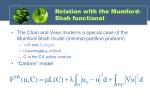Relation with the Mumford-Shah functional
• The Chan and Vese model is a special case of the Mumford Shah model (minimal partition problem)
• =0 and 1=2=
• u=average(u0 in/out)
• C is the CV active contour
• “Cartoon” modelLevel set formulation
• Considering the disadvantages of the active contour representation the model is solved using level set formulation
• level set form -> no explicit contourReplacing C with Φ
• Introducing the Heaviside (sign) and Dirac (PSF) functionsReplacing C with Φ
• The intensity termsAverage intensities
• We can calculate the average intensities using the step functionLevel set formulation of the model

Combining the above presented energy terms we can write the Chan and Vese functional as a function of Φ.

Minimization F wrt. Φ -> gradient descent

The corresponding Euler-Lagrange equation:The algorithm
• Initialization n=0
• repeat
• n++
• Computing c1 and c2
• Evolving the level-set function
• until the solution is stationary, or n>nmaxInitialization
• We set the values of the level set function
• outside = -1
• inside = 1
• Any shape can be the initialization shape

init()

for all (x, y) in Phi

if (x, y) is inside

Phi(x, y)=1;

else

Phi(x, y)=-1;

fi;

end forComputing c1 and c2
• The mean intensity of the image pixels inside and outside

colors()

out = find(Phi < 0);

in = find(Phi > 0);

c1 = sum(Img(in)) / size(in);

c2 = sum(Img(out)) / size(out);Finite differences

for all (x, y)

fx(x, y) = (Phi(x+1, y)-Phi(x-1, y))/(2*delta_s);

fy(x, y) =…

fxx(x, y) =…

fyy(x, y) =…

fxy(x, y) =…

delta_s recommended between 0.1 and 1.0Curvature

curvature = (fx.^2.*fyy + fy.^2.*fxx - 2.*fx.*fy.*fxy) ./ (grad.^1.5);

Be careful! Grad can be 0!Force

force = mu * curvature .* gradient_m - nu – lambda1 * (image - c1).^2 + lambda2 * (image - c2).^2;

We should normalize the force. abs(force) <= 1!

Main step:

Phi=Phi+deltaT*force;

deltaT is recommended between 0.01 and 0.9. Be careful deltaT<1!Narrow band

It is useful to compute the level set function not on the whole image domain but in a narrow band near to the contour. Abs()<d

Decreasing the computational complexity.Narrow band
• Initialization n=0
• repeat
• n++
• Determination of the narrow band
• Computing c1 and c2
• Evolving the level-set function on the narrow band
• Re-initialization
• until the solution is stationary, or n>nmaxRe-initialization
• Optional step

H is a normalizing term recommended between 0.1 and 2.

deltaT time step see above!Stop criteria
• Stop the iterations if:
• The maximum iteration number were reached
• Stationary solution:
• The energy is not changing
• The contour is not movingDemonstration of the program
• Thanks for your attentionMATLAB tutorial